Summary of Week 5
1. Cohen and Coon Method: another method for tuning PID control parameters.
1. Under manual control, generate a process reaction curve by introducing a step change in the manipulated variable.
2. From the reaction curve, determine the process gain, K, the time constant, t, and the apparent time delay, tdelay. Using these values, determine prescribed controller parameters.
1. Laplace Transform: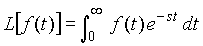Motivation: to transform a function in time into a corresponding function in Laplace variable s, with the expectation that in the new domain, the usual algebraic operations such as multiplication, division, addition and subtraction will make analysis and design of dynamic systems simpler.

Procedure:
1. Given f(t), multiply this first with exp(-st).
2. Then integrate this product with respect to time from t = 0 to t = infinity; .
3. Simplify the integral, e.g. using integration by parts, etc., until you obtain a function of s.
(Note: s is a complex variable having a positive real part.)

Tables of Useful Functions:
 Function Description f (t) L[f (t)] Step Function m(t) 1/s Impulse Function d(t) 1 Ramp Function t 1/s2 Sine Function sin(wt) w/(s2+w2) Cosine Function cos(wt) s/(s2+w2) Exponential Function exp(-a t) 1/(s+a) Power Function tN N!/sN+1

Important Properties:
• Linearity:
L[af(t)+bg(t)] = aL[f(t)] + bL[g(t)]
• Nth Order Derivative: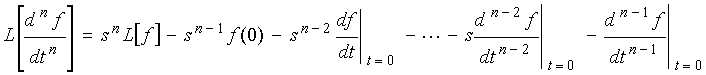• Integral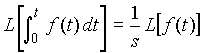• First Shifting Theorem: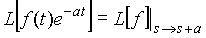• Second Shifting Theorem: Let f(t)=0 for t<0,
L[ f (t - tdelay) ] = exp( - tdelay s ) L[ f ( t ) ]
Solution of Linear Differential Equations:
• Given : A linear differential equation of x(t) with all the necessary initial conditions.
• Procedure:
1. Take Laplace transform of both sides of the differential equation
2. Substitute given initial conditions where needed.
3. Rearrange the equation so that x(s) is equal to a rational polynomial function in s, i.e. a function whose numerator and denominator are polynomials in s.

4. (Note: the order of the numerator polynomial should be smaller than the order of the denominator polynomial.)
5. Use the method of partial fractions to separate x(s) into various terms in which the table of Laplace transforms can be used to obtain the inverse transform.
6. Perform the inverse transforms. (Simplify if desired).

Method of Partial Fractions:

Given: G(s) = num(s)/den(s), where num(s) is the numerator polynomial in s of order N and den(s) is the denominator polynomial in s of order M.

1. Factor den(s): find the M roots of the den(s), e.g. r1, r2,  ,rM.
1. For each factor in the real and distinct roots, say -r, introduce the term A/(s+r), where
2. For each root, say -r, appearing k times, introduce k terms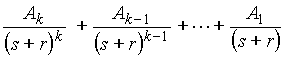1. For each pair of complex conjugate roots:  a + ib and a - ib, introduce two terms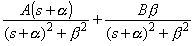1. (Other steps to follow next week).